# (Solved) : Objectives Reading Understanding Code Passes Lists Argument Practice Designing Functions T Q41343879 . . .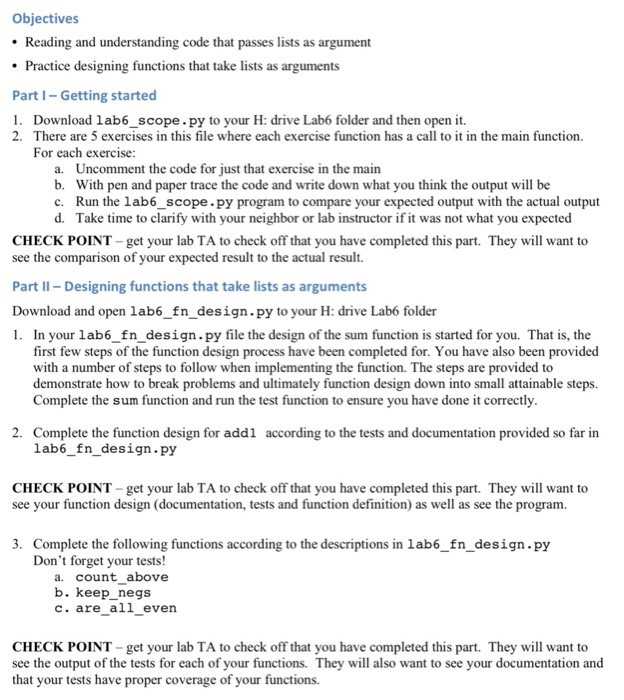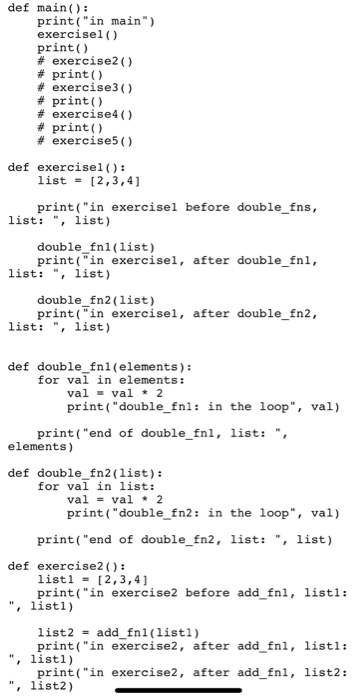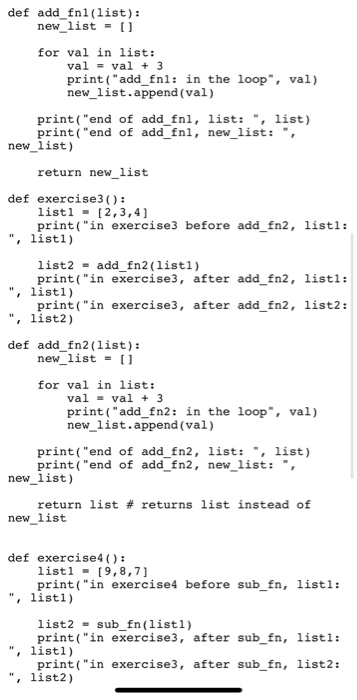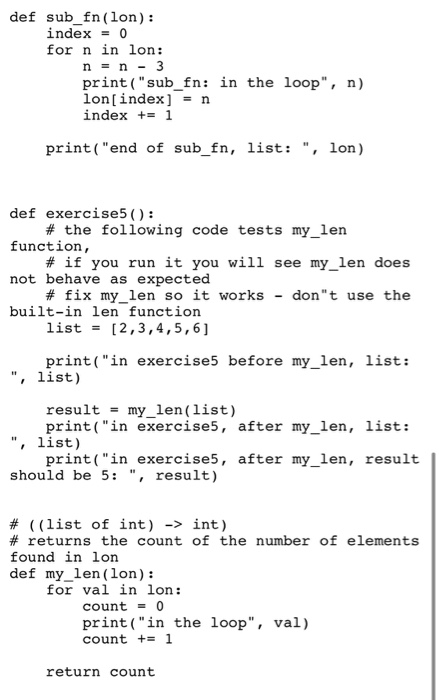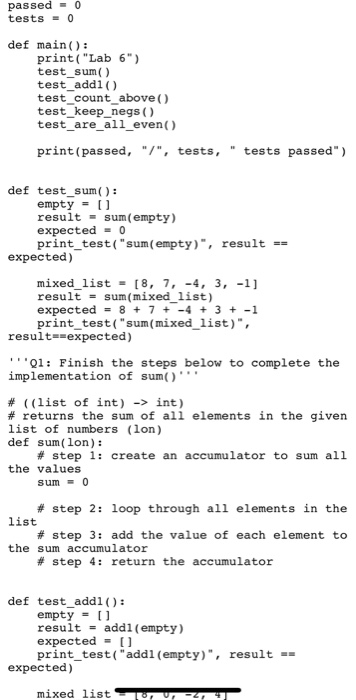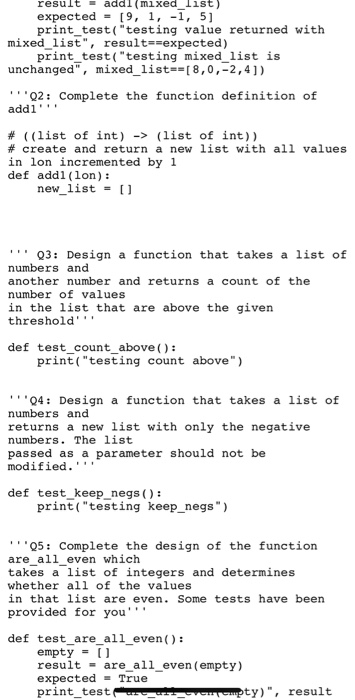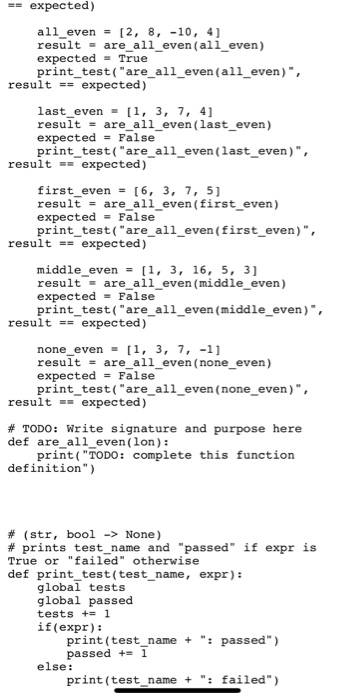Objectives • Reading and understanding code that passes lists as argument • Practice designing functions that take lists as arguments Part I – Getting started 1. Download lab6_scope.py to your H: drive Lab6 folder and then open it. 2. There are 5 exercises in this file where each exercise function has a call to it in the main function. For each exercise: a. Uncomment the code for just that exercise in the main b. With pen and paper trace the code and write down what you think the output will be c. Run the lab6_scope.py program to compare your expected output with the actual output d. Take time to clarify with your neighbor or lab instructor if it was not what you expected CHECK POINT – get your lab TA to check off that you have completed this part. They will want to see the comparison of your expected result to the actual result. Part 1 – Designing functions that take lists as arguments Download and open lab6_fn_design.py to your H: drive Lab6 folder 1. In your lab6_fn_design.py file the design of the sum function is started for you. That is, the first few steps of the function design process have been completed for. You have also been provided with a number of steps to follow when implementing the function. The steps are provided to demonstrate how to break problems and ultimately function design down into small attainable steps. Complete the sum function and run the test function to ensure you have done it correctly. 2. Complete the function design for addi according to the tests and documentation provided so far in lab6_fn_design.py CHECK POINT – get your lab TA to check off that you have completed this part. They will want to see your function design (documentation, tests and function definition) as well as see the program. 3. Complete the following functions according to the descriptions in lab6_fn_design.py Don’t forget your tests! a. count_above b. keep_negs c. are_all_even CHECK POINT – get your lab TA to check off that you have completed this part. They will want to see the output of the tests for each of your functions. They will also want to see your documentation and that your tests have proper coverage of your functions. def main(): print(“in main”) exercisel() print # exercise2() # print # exercise3 # print #exercise4 #print() # exercises def exercisel(): list = [2,3,4] print(“in exercisel before double_fns, list: “, list) double_fni(list) print(“in exercisel, after double_fni, list: “, list) double_fn2(list) print(“in exercisel, after double_fn2, list: “, list) def double_fni(elements): for val in elements: val = val # 2 print(“double_fni: in the loop”, val) print(“end of double_fnl, list: elements) def double_fn2(list): for val in list: val = val + 2 print(“double_fn2: in the loop”, val) print(“end of double_fn2, list: “, list) def exercise2(): listl – [2,3,4] print(“in exercise2 before add_fni, listi: “, listi) list2 = add_fni(listi) print(“in exercise2, after add_fni, listi: “, listi) print(“in exercise2, after add_fni, list2: list2) def add_fni(list): new_list = 0 for val in list: val = val + 3 print(“add fnl: in the loop”, val) new_list.append(val) print(“end of add_fni, list: “, list) print(“end of add_fni, new_list: new_list) return new_list def exercise3(): listl – [2,3,4] print(“in exercise3 before add_fn2, listi: “, listi) list2 = add_fn2(listi) print(“in exercise3, after add_fn2, listi: “, listi) print(“in exercise3, after add_fn2, list2: ” list2) def add_fn2(list): new_list = 0 for val in list: val = val + 3 print(“add_fn2: in the loop, val) new_list.append(val) print(“end of add fn2, list: , list) print(“end of add_fn2, new_list: new_list) return list # returns list instead of new_list def exercise4(): listl – [9,8,7] print(“in exercise4 before sub_fn, listi: “, listi) list 2 – sub_fn(listi) print(“in exercise3, after sub_fn, listi: “, listi) print(“in exercise3, after sub_fn, list2: “, list2) def sub_fn(lon): index=0 for n in lon: n = n – 3 print(“sub_fn: in the loop”, n) lon[index] = n index += 1 print(“end of sub_fn, list: “, lon) def exercise5(): # the following code tests my_len function, # if you run it you will see my_len does not behave as expected # fix my_len so it works – don’t use the built-in len function list = [2,3,4,5,6] print(“in exercise5 before my_len, list: “, list) result = my len(list) print(“in exercises, after my_len, list: “, list) print(“in exercises, after my_len, result should be 5: “, result) # ((list of int) -> int) # returns the count of the number of elements found in lon def my_len (lon): for val in lon: count = 0 print(“in the loop”, val) count += 1 return count passed = 0 tests = 0 def main(): print(“Lab 6”) test_sum test_addi() test_count_above() test_keep_negs() test_are_all_even() print(passed, “/”, tests, ” tests passed”) def test_sum(): empty = 0 result = sum(empty) expected – 0 print_test(“sum (empty)”, result == expected) mixed_list = [8, 7, -4, 3, -1] result = sum(mixed_list) expected = 8 + 7 + – 4 + 3 + -1 print_test(“sum(mixed_list)”, result==expected) ”’01: Finish the steps below to complete the implementation of sum() # ((list of int) -> int) # returns the sum of all elements in the given list of numbers (lon) def sum(lon): # step 1: create an accumulator to sum all the values sum = 0 # step 2: loop through all elements in the list # step 3: add the value of each element to the sum accumulator # step 4: return the accumulator def test_addi(): empty = 0 result = addl (empty) expected = 0 print_test(“addl (empty)”, result == expected) mixed listu PZ result – aaal (mixed_list) expected = [9, 1, -1, 5] print_test(“testing value returned with mixed_list”, result==expected) print_test(“testing mixed_list is unchanged”, mixed_list==[8,0,-2,4]) Q2: Complete the function definition of addi” # ((list of int) -> (list of int)) # create and return a new list with all values in lon incremented by 1 def addl (lon): new_list = [] “” 03: Design a function that takes a list of numbers and another number and returns a count of the number of values in the list that are above the given threshold’! def test_count_above(): print(“testing count above”) ”04: Design a function that takes a list of numbers and returns a new list with only the negative numbers. The list passed as a parameter should not be modified.’ def test keep negs(): print(“testing keep_negs”) ”25: Complete the design of the function are_all_even which takes a list of integers and determines whether all of the values in that list are even. Some tests have been provided for you” def test_are_all_even(): empty = 0 result – are_all_even(empty) expected = True print_test C ompty)”, result == expected) all_even = {2, 8, -10, 4) result = are all even(all even) expected = True print_test(“are_all_even(all_even)”, result == expected) last_even = [1, 3, 7, 4] result = are_all_even(last_even) expected = False print_test(“are_all_even (last_even)”, result == expected) first_even = [6, 3, 7, 5] result = are_all_even (first_even) expected = False print_test(“are_all_even(first_even)”, result == expected) middle_even = (1, 3, 16, 5, 3] result = are all even (middle even) expected = False print_test(“are_all_even (middle_even)”, result == expected) none_even = [1, 3, 7, -1] result = are_all_even (none_even) expected = False print_test(“are_all_even (none_even)”, result == expected) # TODO: Write signature and purpose here def are_all_even(lon): print(“TODO: complete this function definition”) # (str, bool -> None) # prints test_name and passed if expr is True or “failed” otherwise def print_test(test_name, expr): global tests global passed tests += 1 if (expr): print(test_name + “: passed”) passed + 1 else: print(test_name + “: failed”) Show transcribed image text Objectives • Reading and understanding code that passes lists as argument • Practice designing functions that take lists as arguments Part I – Getting started 1. Download lab6_scope.py to your H: drive Lab6 folder and then open it. 2. There are 5 exercises in this file where each exercise function has a call to it in the main function. For each exercise: a. Uncomment the code for just that exercise in the main b. With pen and paper trace the code and write down what you think the output will be c. Run the lab6_scope.py program to compare your expected output with the actual output d. Take time to clarify with your neighbor or lab instructor if it was not what you expected CHECK POINT – get your lab TA to check off that you have completed this part. They will want to see the comparison of your expected result to the actual result. Part 1 – Designing functions that take lists as arguments Download and open lab6_fn_design.py to your H: drive Lab6 folder 1. In your lab6_fn_design.py file the design of the sum function is started for you. That is, the first few steps of the function design process have been completed for. You have also been provided with a number of steps to follow when implementing the function. The steps are provided to demonstrate how to break problems and ultimately function design down into small attainable steps. Complete the sum function and run the test function to ensure you have done it correctly. 2. Complete the function design for addi according to the tests and documentation provided so far in lab6_fn_design.py CHECK POINT – get your lab TA to check off that you have completed this part. They will want to see your function design (documentation, tests and function definition) as well as see the program. 3. Complete the following functions according to the descriptions in lab6_fn_design.py Don’t forget your tests! a. count_above b. keep_negs c. are_all_even CHECK POINT – get your lab TA to check off that you have completed this part. They will want to see the output of the tests for each of your functions. They will also want to see your documentation and that your tests have proper coverage of your functions.
def main(): print(“in main”) exercisel() print # exercise2() # print # exercise3 # print #exercise4 #print() # exercises def exercisel(): list = [2,3,4] print(“in exercisel before double_fns, list: “, list) double_fni(list) print(“in exercisel, after double_fni, list: “, list) double_fn2(list) print(“in exercisel, after double_fn2, list: “, list) def double_fni(elements): for val in elements: val = val # 2 print(“double_fni: in the loop”, val) print(“end of double_fnl, list: elements) def double_fn2(list): for val in list: val = val + 2 print(“double_fn2: in the loop”, val) print(“end of double_fn2, list: “, list) def exercise2(): listl – [2,3,4] print(“in exercise2 before add_fni, listi: “, listi) list2 = add_fni(listi) print(“in exercise2, after add_fni, listi: “, listi) print(“in exercise2, after add_fni, list2: list2)